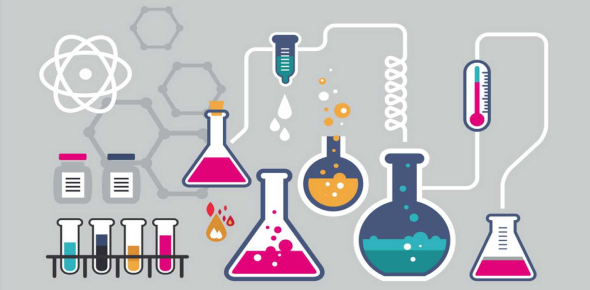# Chemical Reaction And Equation Quiz!

30 Questions | Total Attempts: 1283SettingsCreate your own Quiz.

• 1.
Chemically rust is:
• A.

Hydrated ferrous oxide

• B.

Only ferric oxide

• C.

Hydrated ferric oxide

• D.

None of these

• 2.
Both CO2 and H2 gases are:
• A.

Heavier than air

• B.

Colourless

• C.

Acidic in nature

• D.

Soluble in water

• 3.
Fatty food becomes rancid due to the process of:
• A.

Oxidation

• B.

Corresion

• C.

Reduction

• D.

Hydrogenetion

• 4.
We store silver chloride in a dark-colored bottle because it is:
• A.

A white solid

• B.

Undergoes redox reaction

• C.

To avoid action of sunlight

• D.

None of these

• 5.
When a magnesium ribbon is burnt in the air, the ash formed is:
• A.

Black

• B.

White

• C.

Yellow

• D.

Pink

• 6.
In which of the following heat energy will be evolved?
• A.

Electrolysis of water

• B.

Dissolution of NH4Cl in water

• C.

Burning of L.P.G.

• D.

Decomposition of AgBr in presence of sunlight

• 7.
Rancidity can be prevented by:
• A.

• B.

Storing food away from the light

• C.

Keeping food in refrigeretor

• D.

All of these

• 8.
The reaction of H2 gas with oxygen gas to form water is an example of:
• A.

Combination reaction

• B.

Redox reaction

• C.

Exothermic reaction

• D.

All of these reaction

• 9.
The reaction in which two compound exchange their ions to form two new compounds is called:
• A.

Displacement reaction

• B.

Combination reaction

• C.

Double displacement reaction

• D.

Redox reaction

• 10.
An element X on exposure to moist air turns reddish-brown and a new compound Y is formed. The substance X and Y are
• A.

X=Fe, Y=Fe2O2

• B.

X=Ag, Y=Ag2s

• C.

X=Cu, Y=CuO

• D.

X=Al, Y=Al2O3

• 11.
Before burning in air, the magnesium ribbon is cleaned by rubbing with a sandpaper to:
• A.

Make the ribbon surface shinier

• B.

Remove the layer of magnesium oxide from the ribbon surface

• C.

Remove the layer of magnesium carbonate from the ribbon surface

• D.

Remove the moisture from the ribbon surface

• 12.
In a chemical reaction between sulphuric acid and barium chloride solution the white precipitates formed are of:
• A.

Hydrochloric acid

• B.

Barium sulphate

• C.

Chlorine

• D.

Sulphur

• 13.
The respiration process during which glucose undergoes slow combustion by combining with oxygen in the cells of our body to produce energy is a kind of:
• A.

Exothermic process

• B.

Endothermic process

• C.

Reversible

• D.

Physical process

• 14.
A chemical reaction does not involve:
• A.

Formation of new substances having entirely different properties than that of the  reactants

• B.

Breaking of old chemical bonds and formation of new chemical bonds

• C.

Rearrangement of the atoms of reactants to form new products

• D.

Changing of the atoms of on element into those of another element to form new  products

• 15.
One of the following processes does not involve a chemical reaction. That is:
• A.

Melting of candle wax when heated

• B.

Burning of candle wax when heated

• C.

Digestion of food in our stomach

• D.

Ripening of banana

• 16.
It is necessary to balance a chemical equation in order to satisfy the law of:
• A.

Conservation of motion

• B.

Conservation of momentum

• C.

Conservation of energy

• D.

Conservation of mass

• 17.
You are given the following chemical reaction, this reaction represent:
• A.

Combination reaction as well as double displacement reaction

• B.

Redox reaction as well as displacement reaction

• C.

Double displacement reaction as well as redox reaction

• D.

Decomposition reaction as well as displacement reaction

• 18.
When ferrous sulfate is heated strongly it undergoes decomposition to form ferric oxide as a main product accompanied by a change in colour from:
• A.

Blue to green

• B.

Green to Blue

• C.

Green to Broun

• D.

Broun to Green

• 19.
Which of the following gases is used in the storage of fat and oil-containing foods for a long time?
• A.

Carbondioxide gas

• B.

Nitrogen

• C.

Oxygen

• D.

Neon

• 20.
Following is given a diagram showing an experimental set-up, The given set-up is used to carry out:
• A.

Distillation of water

• B.

Purification of water

• C.

Electrolysis of water

• D.

Hydrolysis

• 21.
The displacement reaction between iron (III) oxide and a metal X is used for welding the rail tracks. Here X is:
• A.

Copper granules

• B.

Magnesium ribbon

• C.

Sodium pellets

• D.

Aluminium dust

• 22.
The neutralization reaction between an acid and a base is a type of:
• A.

Double displacement reaction

• B.

Displacement reaction

• C.

• D.

Decomposition reaction

• 23.
The chemical reaction between Hydrogen sulfide and iodine to give Hydrogen iodide and sulfur is given below, The reducing and oxidizing agents involved in this redox reaction are:
• A.

Iodine and sulphur respectively

• B.

Iodine and hydrogen sulphide respectively

• C.

Sulphur and iodine respectively

• D.

Hydrogen sulphide and sulphur

• 24.
Pb + CuCl2 → PbCl2 + Cu The above reaction is an example of:
• A.

Combination

• B.

Double displacement

• C.

Decomposition

• D.

Displacement

• 25.
Most reactive metal is:
• A.

Mg

• B.

Na

• C.

K

• D.

Al

Related TopicsBack to top
×

Wait!
Here's an interesting quiz for you.